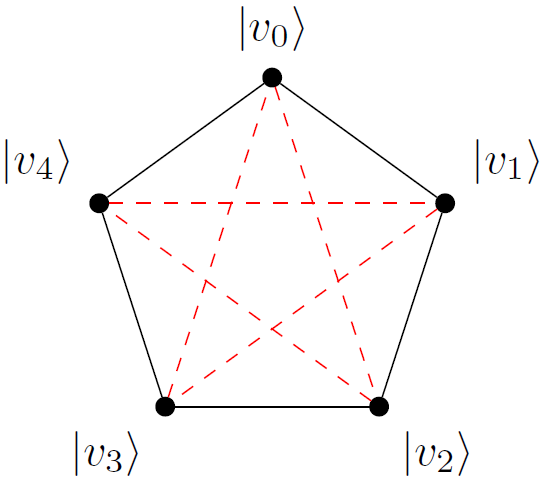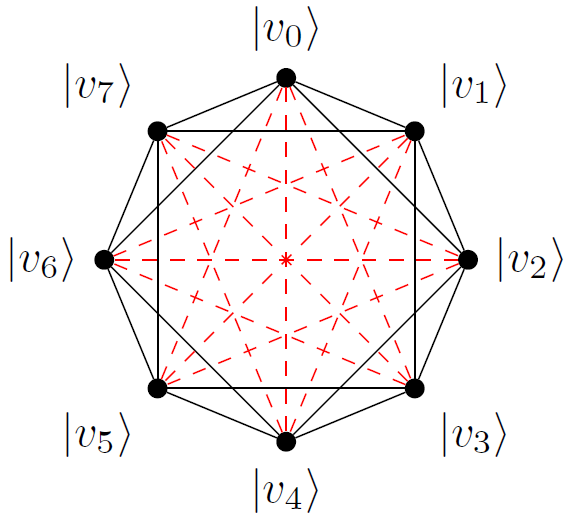Blog > How to Construct Minimal Unextendible Product Bases

## How to Construct Minimal Unextendible Product Bases

March 14th, 2013

In quantum information theory, a product state$|v\rangle \in \mathbb{C}^{d_1}\otimes\mathbb{C}^{d_2}$ is a quantum state that can be written as an elementary tensor:$|v\rangle=|v_1\rangle\otimes|v_2\rangle\text{ with }|v_i\rangle\in\mathbb{C}^{d_i}\ \text{ for } i=1,2,$

while states that can not be written in this form are called entangled. In this post, we will be investigating unextendible product bases (UPBs), which are sets$S\subset\mathbb{C}^{d_1}\otimes\mathbb{C}^{d_2}$ of mutually orthogonal product states with the property that no other product state is orthogonal to every member of$S$.

In this post, we will be looking at how to construct small UPBs. Note that UPBs can more generally be defined on multipartite spaces (i.e.,$\mathbb{C}^{d_1}\otimes\mathbb{C}^{d_2}\otimes\cdots\otimes\mathbb{C}^{d_p}$ for arbitrary$p\geq 2$), but for simplicity we stick with the bipartite (i.e.,$p= 2$) case in this blog post.

### Simple Examples

The most trivial unextendible product basis is simply the computational basis:$S:=\big\{|0\rangle\otimes|0\rangle,\ldots,|0\rangle\otimes|d_2-1\rangle,\ldots,|d_1-1\rangle\otimes|0\rangle,\ldots,|d_1-1\rangle\otimes|d_2-1\rangle\big\}.$

However, the above UPB is rather trivial – the unextendibility condition holds vacuously because$S$ spans the entire Hilbert space, so of course there is no product state (or any state) orthogonal to every member of$S$.

It is known that when$\min\{d_1,d_2\}\leq 2$, the only UPBs that exist are trivial in this sense – they consist of a full set of$d_1d_2$ states. We are more interested in UPBs that contain fewer vectors than the dimension of the Hilbert space (since, for example, these UPBs can be used to construct bound entangled states ). One of the first such UPBs to be constructed was called “Pyramid” . To construct this UPB, define$h:=\tfrac{1}{2}\sqrt{1+\sqrt{5}}$ and$N:=\tfrac{1}{2}\sqrt{5+\sqrt{5}}$, and let$|\phi_j\rangle:=\tfrac{1}{N}[\cos(2\pi j/5),\sin(2\pi j/5),h]\text{ for }0\leq j\leq 4.$

Then the following set of 5 states in$\mathbb{C}^3\otimes\mathbb{C}^3$ is a UPB:$S_{\textup{pyr}}:=\big\{|v_0\rangle,|v_1\rangle,|v_2\rangle,|v_3\rangle,|v_4\rangle\big\},$

where$|v_i\rangle:=|\phi_i\rangle\otimes|\phi_{2i(\text{mod }5)}\rangle$.

It is a straightforward calculation to verify that the members of$S_{\textup{pyr}}$ are mutually orthogonal (and thus form a product basis). To verify that there is no product state orthogonal to every member of$S_{\textup{pyr}}$, we first observe that any 3 of the$|\phi_j\rangle$‘s form a linearly independent set (verification of this claim is slightly tedious, but nonetheless straightforward). Thus there is no state$|w\rangle\in\mathbb{C}^3$ that is orthogonal to more than 2 of the$|\phi_j\rangle$‘s. Thus no product state$|w_1\rangle\otimes|w_2\rangle\in\mathbb{C}^3\otimes\mathbb{C}^3$ is orthogonal to more than 2 + 2 = 4 members of$S_{\textup{pyr}}$, which verifies unextendibility.

### Minimum Size

One interesting question concerning unextendible product bases asks for their minimum cardinality. It was immediately noted that any UPB in$\mathbb{C}^{d_1}\otimes\mathbb{C}^{d_2}$ must have cardinality at least$d_1+d_2-1$. To see this, suppose for a contradiction that there existed a UPB$S$ containing$(d_1-1)+(d_2-1)$ or fewer product states. Then we could construct another product state that is orthogonal to$d_1-1$ members of$S$ on$\mathbb{C}^{d_1}$ and another$d_2-1$ members of$S$ on$\mathbb{C}^{d_2}$, for a total of$(d_1-1)+(d_2-1)$ members of$S$, which shows that$S$ is extendible.

Despite being such a simple lower bound, it is also attainable for many values of$d_1,d_2$  (and very close to attainable in the other cases [3,4]). The goal of this post is to focus on the case when there exists a UPB of cardinality$d_1+d_2-1$, which is characterized by the following result of Alon and Lovász:

Theorem . There exists a UPB in$\mathbb{C}^{d_1}\otimes\mathbb{C}^{d_2}$ of (necessarily minimal) size$d_1+d_2-1$ if and only if$d_1,d_2\geq 3$ and at least one of$d_1$ or$d_2$ is odd.

In spite of the above result that demonstrates the existence of a UPB of the given minimal size in many cases, how to actually construct such a UPB in these cases is not immediately obvious, and is buried throughout the proofs of  and its references. The goal of the rest of this post is to make the construction of a minimal UPB in these cases explicit.

### Orthogonality Graphs

The orthogonality graph of a set of$s$ product states in$\mathbb{C}^{d_1}\otimes\mathbb{C}^{d_2}$ is graph with coloured edges (there are 2 colours) on$s$ vertices (one for each product state), such that there is an edge connecting two vertices with the$i$th colour if and only if the two corresponding product states are orthogonal on the$i$th party.

For example, the orthogonality graph of the Pyramid UPB introduced earlier is illustrated below. Black edges represent states that are orthogonal on the first party, and red dotted edges represent states that are orthogonal on the second party.If the product states under consideration are mutually orthogonal, then their orthogonality graph is the complete graph$K_s$. Unextendibility is a bit more difficult to determine, but nonetheless a useful technique for constructing UPBs is to first choose a colouring of the edges of$K_s$, and then try to construct product states that lead to that colouring.

### A Minimal Construction

In the orthogonality graph of the Pyramid UPB, all of the edges that connect a vertex to a neighbouring vertex are coloured black, and all other edges are coloured red. We can construct minimal UPBs by generalizing this graph in a natural way. Suppose without loss of generality that$d_1$ is odd, and we wish to construct a UPB of size$s := d_1 + d_2 - 1$. We construct the orthogonality graph by arranging$s$ vertices in a circle and connecting any vertices that are a distance of$(d_1-1)/2$ or less from each other via a black edge. All other edges are coloured red. For example, in the$d_1 = d_2 = 3$ case, this gives the orthogonality graph above. In the$d_1 = 5, d_2 = 4$ case, this gives the orthogonality graph below.Our goal now is to construct product states that have the given orthogonality graph. This is straightforward to do, since every state must be orthogonal to$d_1-1$ of the other states on$\mathbb{C}^{d_1}$ and orthogonal to the$d_2-1$ other states on$\mathbb{C}^{d_2}$. Thus, we can just pick$|v_0\rangle$ arbitrarily, then pick$|v_1\rangle$ randomly subject to the constraint that it is orthogonal to$|v_0\rangle$ on the first subsystem, and so on, working our way clockwise around the orthogonality graph, generating each product state randomly subject to the orthogonality conditions.

Furthermore, it can be shown (but will not be shown here – the techniques are similar to those of  and are a bit technical) that this procedure leads to a product basis that is in fact unextendible with probability 1. In order to verify unextendibility explicitly, one approach is to check that any subset of$d_1$ of the product states are linearly independent on$\mathbb{C}^{d_1}$ and any subset of$d_2$ of the product states are linearly independent on$\mathbb{C}^{d_2}$.

References

1. C. H. Bennett, D. P. DiVincenzo, T. Mor, P. W. Shor, J. A. Smolin, and B. M. Terhal. Unextendible product bases and bound entanglement. Phys. Rev. Lett., 82:5385–5388, 1999. E-print: arXiv:quant-ph/9808030
2. N. Alon and L. Lovász. Unextendible product bases. J. Combinatorial Theory, Ser. A, 95:169–179, 2001.
3. K. Feng. Unextendible product bases and 1-factorization of complete graphs. Discrete Appl. Math., 154:942–949, 2006.
4. J. Chen and N. Johnston. The minimum size of unextendible product bases in the bipartite case (and some multipartite cases). Comm. Math. Phys., 333(1):351–365, 2015. E-print: arXiv:1301.1406 [quant-ph]